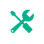# HCF & LCM Calculator

How it Works?
1. 1. Enter numbers in first input box separated by comma
2. 2. Click on calculate button
HCF of
--
Show steps

LCM of
--
Show steps

## LCM Calculator

The online LCM calculator can find the least common multiple (factors) quickly than manual methods. Whether finding the LCM of two numbers or multiple numbers, this calculator can help you with just a single click.Related Tool Name

HCF (GCF) and LCM calculator is used to find the highest common factor and least common multiple at one place. These values are often required together, this is why this calculator provides both at the same place.

The HCF finder shows steps for both HCF and LCM calculations. You can see the manual process of calculation for HCF and LCM in three different methods. Common factors are also highlighted.

## What is HCF?

HCF, as you may already know, stands for Highest Common Factor. It is the highest value factor that is common for values under consideration.

It is to be noted that HCF and GCF are different names for the same thing. It is also called GCD, the greatest common divisor.

## What is LCM?

LCM is the abbreviation for the least common factor. It is the opposite of HCF. It is the lowest value multiple that is common for the values under study.

Now that you know the basic definitions, let’s see the methods to find HCF and LCM.

## How to find HCF and LCM?

There are three main methods used to find the HCF or LCM for two or more numbers. These methods are:

• Prime Factorization
• Division method
• List of factors/multiples

The most common method is prime factorization. Let’s see an example of this method.

### Example:

Find the HCF of 16, 32. Use the Prime factorization method.

### Solution:

Step 1: Break down into prime factors.

16 = 2 x 2 x 3 = 22 x 3

32 = 2 x 2 x 2 x 2 = 24

Step 2: Identify similar factors.

Both numbers have 22 as their factors.

Step 3: Multiply the common factors.

22 = 2 x 2 = 4

Hence 4 is the HCF of 16 and 32